Successfully reported this slideshow.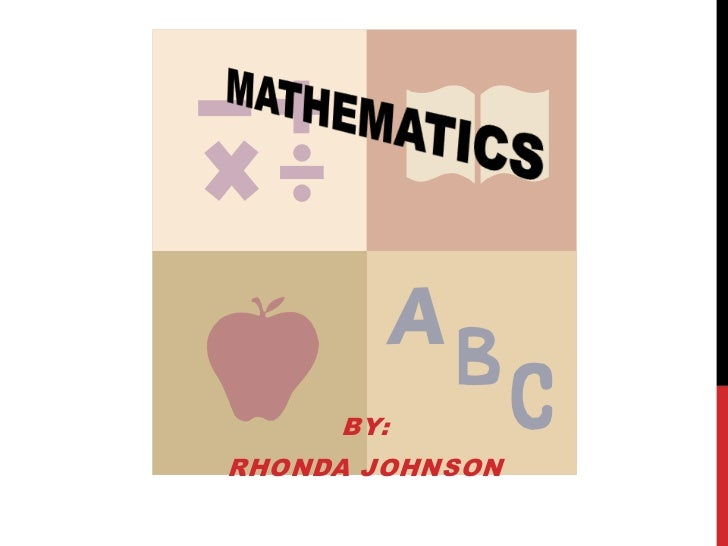Upcoming SlideShare
×

of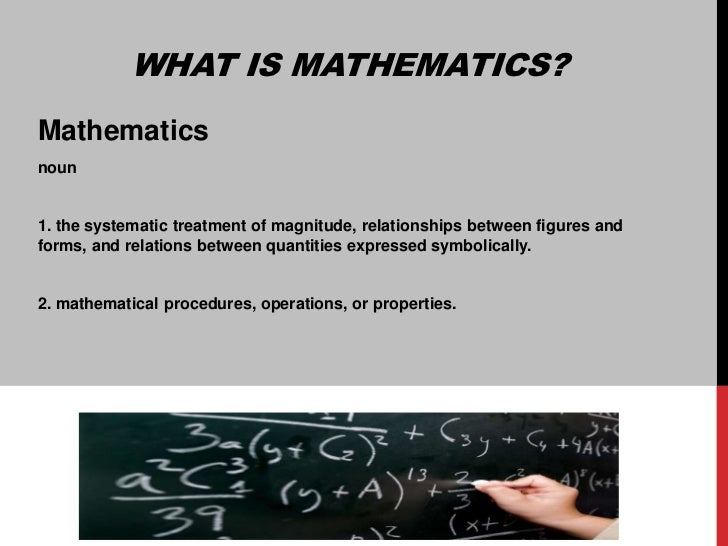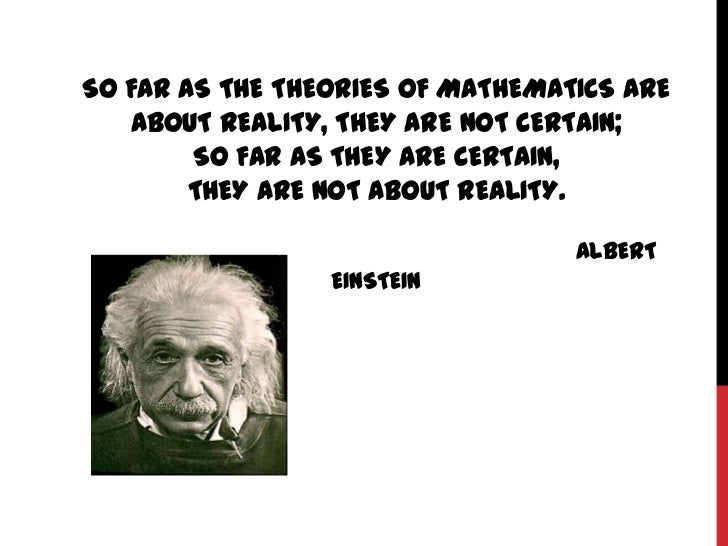Upcoming SlideShare
Math Powerpoint Final 1
Next

26 Likes

Share

# Mathematics

See all

See all

### Mathematics

1. 1. MATHEMATICS<br />By:<br />Rhonda Johnson<br />
2. 2. What is mathematics?<br />Mathematics<br />noun <br />1. the systematic treatment of magnitude, relationships between figures and forms, and relations between quantities expressed symbolically. <br />2. mathematical procedures, operations, or properties.<br />
3. 3. So far as the theories of mathematics are about reality, they are not certain; so far as they are certain, they are not about reality. Albert Einstein<br />
4. 4. Why Do People Hate Math?<br />The way math was taught to them.<br />They do not understand the concepts of math.<br />Math is boring and too hard to learn.<br />Too much homework and studying.<br />Too many formulas and equations to remember.<br />Confusing.<br />Math is not needed beyond schooling.<br />I will never need to know this Math when I am older.<br />
5. 5. Different Types Of Math<br />
6. 6. Algebra : the branch of Mathematics concerning the study of the rules of Operations and Relations, and the constructions and concepts arising from them, including terms, polynomials Equations and Algebraic Structures. Together with Geometry, analysis, topology, combinatorics, and number theory, algebra is one of the main branches of pure mathematics.<br />Example: 3x+24=8+5x <br /> (3x+24)-3x=8+5x-3<br /> 24=8+2x<br /> 24=(8+2x)-8<br /> 24-8=2x<br /> 16=2x<br /> 16/2=2x/2<br /> 8=x<br /> x=8<br />
7. 7. Geometry:a branch of Mathematics concerned with questions of shape, size, relative position of figures, and the properties of space. Geometry is one of the oldest mathematical sciences. <br />
8. 8. Trigonometry:the computational component of geometry. For instance, one statement of plane geometry states that a triangle is determined by a side and two angles. In other words, given one side of a triangle and two angles in the triangle, then the other two sides and the remaining angle are determined. Trigonometry includes the methods for computing those other two sides. The remaining angle is easy to find since the sum of the three angles equals 180 degrees (usually written 180°). <br />Pythagoreantheorem: a2 + b2 = c2. (a theorytoremember)<br />Sines: sin A = a/c, sin B = b/c.<br />Cosines: cosA = b/c,cosB = a/c.<br />Tangents: tan A = a/b, tan B = b/a<br />
9. 9. cALCULUS:a branch of Mathematics focused on LIMITS, FUNCTIONS, DERIVATIVES, INTEGRALS, AND INFINITE SERIES. This subject constitutes a major part of modern MATHEMATICS EDUCATION. It has two major branches, DIFFERENTIAL CALCULUS and INTEGRAL CALCULUS, which are related by the FUNDAMENTAL THEOREM OF CALCULUS. calculus is the study of change, in the same way that Geometry is the study of shape and algebra is the study of operations and their application to solving equations. A course in calculus is a gateway to other, more advanced courses in mathematics devoted to the study of functions and limits, broadly called mathematical analysis. Calculus has widespread applications in science, economics, and engineering and can solve many problems for which algebra alone is insufficient. <br />Calculus Tutorial Online in case you should need help. Also, you can refer to this website of a Professor’s Class Notes.<br />(This math is a little harder to explain and just show some examples. This math is starting to get harder and harder. With some extra studying, practice and help you will survive.)<br />
10. 10. Why do we need to learn math?<br /><ul><li>Learning mathematics forces one to learn how to think very logically and to solve problems using that skill.
11. 11. Math really is important for your daily life, no matter what you want to do. It's true that not everything you learn will be needed later, but you never know what you'll want to do, and the more you know and have to build on, the better off you'll be.
12. 12. In reality math is the basic language of the world, for everyone uses math in most everything they do (often without realizing it).
13. 13. Every subject contains information necessary to become a knowledgeable and functional member of our society</li></li></ul><li>“If you look at it the right way, math is not a chore of memorization, it's a game where you start with a few simple rules and see what more complicated rules you can come up with using those simple tools.”<br /> Author Unknown<br />
14. 14. Life is good for only two things, discovering mathematics and teaching mathematicsSiméonPoisson<br />Mathematics is a game played according to certain rules with meaningless marks on paper. David Hilbert (1862-1943)<br />
15. 15. If you are having trouble in math do not wait until you are behind. Seek help or find a tutor. Maybe you are missing a single step and not getting the answer right the first time.<br />Take a moment to stop and ask for help! This is almost as good as calling 911 for help!<br />
•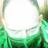#### AliSafaa3

Aug. 23, 2020
•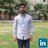#### amnakhan786

Jan. 24, 2020
•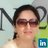#### AzkaQureshi

Aug. 2, 2019
•Jul. 14, 2019
•#### saribshafique

Apr. 22, 2019
•#### Srsourav

Apr. 16, 2019
•#### GnikYrrej

Sep. 25, 2018
•#### Nikhilagrwal

Sep. 10, 2018
•Aug. 31, 2018
•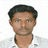#### PrasanthKaruppasamy

Aug. 16, 2018
•#### DEVA85

Aug. 6, 2018
•#### ronilgoyal1

Jun. 17, 2018
•#### RanaInamullahTubassa

Apr. 5, 2018
•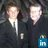#### JosuaWesch

Mar. 1, 2018
•#### hdhdjjudjud

Dec. 4, 2017
•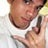#### aphle

Dec. 1, 2017
•#### JacquieFreund

Aug. 1, 2017
•#### IsaacGaitho

Mar. 28, 2016
•#### 042567

Sep. 12, 2015
•Jan. 31, 2015

Total views

42,644

On Slideshare

0

From embeds

0

Number of embeds

304

715

Shares

0